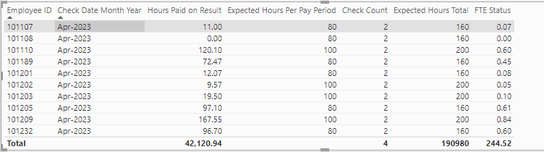cancel
Showing results for
Did you mean:Helper V

## Help with measure - Actual hours worked vs required hours

Hello,

I am trying to calculate each employees percentage of a full-time employee they are working.  By employee, by month, I pull their actual hours worked vs what full-time hours are for that type of employee, and divide to find the percentage of Full-time they are.

For example, I have an Anesthetist that worked total 60 hours in April, based off of 2 paychecks.  A full-time Anesthetist should work 80 hours per paycheck, times 2 paychecks, 160 hours.  So 60/160, this person is .375 Full time employee

Actual hours is given - Hours paid on Result

Expected Hours per paycheck:

Expected Hours Per Pay Period = IF('Per Diem Pay Slips'[Job Family]="CL-Anesthetist", 80, IF('Per Diem Pay Slips'[Job Family]="CL-Anesthesiologist", 100, 0))

Count of Paychecks:
Check Count = DISTINCTCOUNT('Per Diem Pay Slips'[Check Date])

Expected Hours Total:
Expected Hours Total = CALCULATE(MIN('Per Diem Pay Slips'[Expected Hours Per Pay Period]) * [Check Count])

FTE Status = CALCULATE(DIVIDE(SUM('Per Diem Pay Slips'[Hours Paid on Result]), [Expected Hours Total]))

This works on an employee level.  But if I need to see totals, total FTE Status for everybody, I get 0.  See totals at bottom of columns, Expected Hours Total is not summing?  I don't know how to revise my measures.1 ACCEPTED SOLUTIONHelper V

So, it looked like it was doubling or tripling the FTE Status by Employee (multiplying by number of checks):So I took Check Date out of the formula and it looks like it's working now:

FTE Status = SUMX (
SUMMARIZE (
'Per Diem Pay Slips',
'Per Diem Pay Slips'[Employee ID]
),
CALCULATE (
DIVIDE (
SUM ( 'Per Diem Pay Slips'[Hours Paid on Result] ),
[Expected Hours Total]
)
)
)4 REPLIES 4Super User

You need to iterate over a summary table using the same columns as in the table visual, e.g.

``````Expected Hours Total =
SUMX (
SUMMARIZE (
'Per Diem Pay Slips',
'Per Diem Pay Slips'[Employee ID],
'Per Diem Pay Slips'[Check date]
),
CALCULATE (
MIN ( 'Per Diem Pay Slips'[Expected Hours Per Pay Period] ) * [Check Count]
)
)
``````Helper V

Wow, thank you so much, that worked.  But now the FTE Status is not summing, do I have to do something similar?

FTE Status = CALCULATE(DIVIDE(SUM('Per Diem Pay Slips'[Hours Paid on Result]), [Expected Hours Total]))Super User

Yes, its the same pattern

``````FTE Status =
SUMX (
SUMMARIZE (
'Per Diem Pay Slips',
'Per Diem Pay Slips'[Employee ID],
'Per Diem Pay Slips'[Check date]
),
CALCULATE (
DIVIDE (
SUM ( 'Per Diem Pay Slips'[Hours Paid on Result] ),
[Expected Hours Total]
)
)
)
``````Helper V

So, it looked like it was doubling or tripling the FTE Status by Employee (multiplying by number of checks):So I took Check Date out of the formula and it looks like it's working now:

FTE Status = SUMX (
SUMMARIZE (
'Per Diem Pay Slips',
'Per Diem Pay Slips'[Employee ID]
),
CALCULATE (
DIVIDE (
SUM ( 'Per Diem Pay Slips'[Hours Paid on Result] ),
[Expected Hours Total]
)
)
)